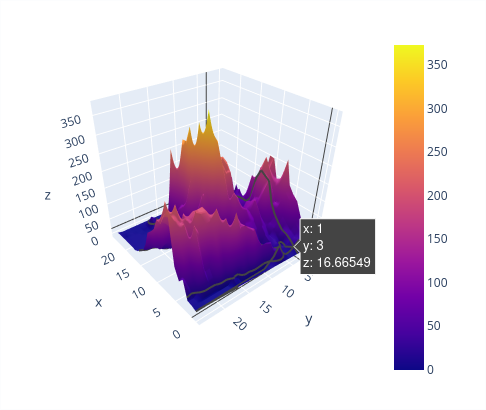# Show plots, charts & graphs

## Using Plotly​

New in v12.10.0

`PlotlyView` for embedding a Plotly graph in a view

Plotly is a free and open source package that simplifies the creation of interactive graphs, such as line plots, scatter plots, histograms, etc. A Plotly graph can be incorporated in your VIKTOR app by making use of a `PlotlyView`.Example of how an interactive visualization in the PlotlyView could look like (source: plot.ly)

The Plotly graph can either be passed to a `PlotlyResult` as a (JSON) `str` representation (e.g. by creating a Plotly `Figure` object and calling `to_json` on it), or as a valid Plotly `dict`:

``import plotly.graph_objects as gofrom viktor import ViktorControllerfrom viktor.views import PlotlyView, PlotlyResultclass Controller(ViktorController):    ...    @PlotlyView("Plotly view", duration_guess=1)    def get_plotly_view(self, params, **kwargs):        fig = go.Figure(            data=[go.Bar(x=[1, 2, 3], y=[1, 3, 2])],            layout=go.Layout(title=go.layout.Title(text="A Figure Specified By A Graph Object"))        )        return PlotlyResult(fig.to_json())``
``from viktor import ViktorControllerfrom viktor.views import PlotlyView, PlotlyResultclass Controller(ViktorController):    ...    @PlotlyView("Plotly view", duration_guess=1)    def get_plotly_view(self, params, **kwargs):        fig = {            "data": [{"type": "bar", "x": [1, 2, 3], "y": [1, 3, 2]}],            "layout": {"title": {"text": "A Figure Specified By Python Dictionary"}}        }        return PlotlyResult(fig)``

A Plotly view can be combined with data using a `PlotlyAndDataView`

### Testing​

New in v13.3.0

`mock_View` decorator for easier testing of view methods

Methods decorated with `@PlotlyView` or `@PlotlyAndDataView` need to be mocked within the context of (automated) testing.

``import unittestfrom viktor.testing import mock_Viewfrom app.my_entity_type.controller import MyEntityTypeControllerclass TestMyEntityTypeController(unittest.TestCase):    @mock_View(MyEntityTypeController)    def test_plotly_view(self):        params = ...        result = MyEntityTypeController().plotly_view(params=params)        self.assertEqual(result.figure, ...)``

## Using Matplotlib​

You can show Matplotlib figures as static images using `ImageView`.

If you want an interactive graph and have a more sophisticated data visualisation tool, we recommend using Plotly.

Here is an example of how to use Matplotlib:

``from viktor import ViktorControllerfrom viktor.parametrization import ViktorParametrization, NumberField, Textfrom viktor.views import ImageResult, ImageViewimport numpy as npimport matplotlib.pyplot as pltfrom io import StringIOclass Parametrization(ViktorParametrization):    introduction = Text("Fill in values for A, B and C to plot the following equation: \$y = Ax^2 + Bx + C\$")    constant_a = NumberField('A',default=1)    constant_b = NumberField('B', default=1)    constant_c = NumberField('C', default=1)class Controller(ViktorController):    label = 'My Entity Type'    parametrization = Parametrization    @ImageView("Plot", duration_guess=1)    def createPlot(self, params, **kwargs):        fig = plt.figure()        x = np.arange(-10, 10, 1)        y = params.constant_a*x**2 + params.constant_b*x + params.constant_c        plt.plot(x, y)        svg_data = StringIO()        fig.savefig(svg_data, format='svg')        plt.close()        return ImageResult(svg_data)``